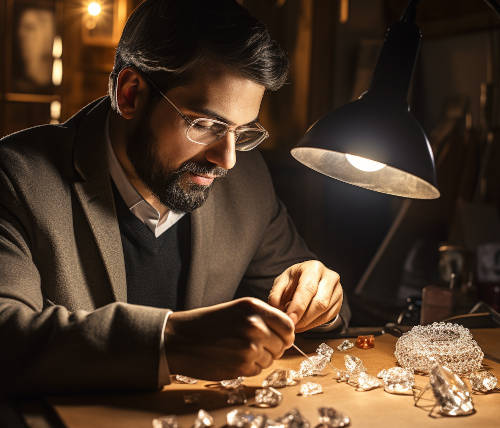# Can the chi squared test detect fake primes?This morning I wrote about Dan Piponi’s fake prime function. This evening I thought about it again and wondered whether the chi-squared test could tell the difference between the distribution of digits in real primes and fake primes.

If the distributions were obviously different, this would be apparent from looking at histograms. When distributions are more similar, visual inspection is not as reliable as a test like chi-squared. For small samples, we can be overly critical of plausible variations. For large samples, statistical tests can detect differences our eyes cannot.

When data fall into a number of buckets, with a moderate number of items expected to fall in each bucket, the chi squared test attempts to measure how the actual counts in each bucket compare to the expected counts.

This is a two-sided test. For example, suppose you expect 12% of items to fall in bucket A and 88% to fall in bucket B. Now suppose you test 1,000 items. It would be suspicious if only 50 items landed in bucket A since we’d expect around 120. On the other hand, getting exactly 120 items would be suspicious too. Getting exactly the expected number is unexpected!

Let’s look a the primes, genuine and fake, less than N = 200. We’ll take the distribution of digits in the list of primes as the expected values and compare to the distribution of the digits in the fake primes.

When I ran this experiment, I got a chi-squared value of 7.77. This is an unremarkable value for a sample from a chi-squared distribution with 9 degrees of freedom. (There are ten digits, but only nine degrees of freedom because if you rule out nine possibilities then digit is determined with certainty.)

The p-value in this case, the probability of seeing a value as large as the one we saw or larger, is 0.557.

Next I increased N to 1,000 and ran the experiment again. Now I got a chi-squared value of 19.08, with a corresponding p-value of 0.024. When I set N to 10,000 I got a chi-squared value of 18.19, with a corresponding p-value of 0.033.

When I used N = 100,000 I got a chi-squared value of 130.26, corresponding to a p-value of 10-23. Finally, when I used N = 1,000,000 I got a chi-squared value of 984.7 and a p-value of 3.4 × 10-206.

In short, the chi-squared test needs a fair amount of data to tell that fake primes are fake. The distribution of digits for samples of fake primes less than a thousand or so is plausibly the same as that of actual primes, as far as the test can distinguish. But the chi-squared values get implausibly large for fake primes up to  100,000.

## One thought on “Can the chi squared test detect fake primes?”

1. Mehmet

This question has some parallels with RNG quality checks. A single test usually not sufficient to detect randomness.